NCERT Exemplar: Atoms and Molecules

# NCERT Exemplar: Atoms and Molecules - Science Class 9

## Multiple Choice Questions

Q.1. Which of the following correctly represents 360 g of water?
(i) 2 moles of H20
(ii) 20 moles of water
(iii) 6.022 × 1023 molecules of water
(iv) 1.2044×1025 molecules of water
Choose the option:
(a) (i)
(b) (i) and (iv)
(c) (ii) and (iii)
(d) (ii) and (iv)
Ans: (d)
Explanation:
Number of moles = Mass of water / Molar mass of water
Number of moles = 360g / 12g/mol
Number of moles = 20
Number of molecules = 20 x 6.022 x 1023 = 1.2044 x 1025 molecules of water
Thus, option (d) is correct.Q.2. Which of the following statements is not true about an atom?
(a) Atoms are not able to exist independently
(b) Atoms are the basic units from which molecules and ions are formed
(c) Atoms are always neutral in nature
(d) Atoms aggregate in large numbers to form the matter that we can see, feel or touch
Ans: (d)

Q.3. The chemical symbol for nitrogen gas is
(a) Ni
(b) N2
(c) N+
(d) N
Ans: (b)
Explanation: Chemical formula of Nitrogen is N but Nitrogen exist in molecule of two ions hence chemical symbol of Nitrogen gas is written as N2.

Q.4. The chemical symbol for sodium is
(a) So
(b) Sd
(c) NA
(d) Na
Ans: (d)
Explanation: Sodium word is derived from Latin word Natrium hence the chemical name of sodium is Na.

Q.5. Which of the following would weigh the highest?
(a) 0.2 mole of sucrose (C12 H22 O11)
(b) 2 moles of CO2
(c) 2 moles of CaCO3
(d) 10 moles of H2O
Ans: (c)
Explanation:
The molecular mass of C₁₂H₂₂O₁₁ is = 12(12) + 22(1) + 11(16) gm = 342 gm
0.2 moles of it weigh = 0.2 x 342 gm = 68.4gm
The molar mass of CO₂ is = 12 + 2(16) gm = 44gm
2 moles of it weigh = 2 x 44 = 88gm
The molar mass of CaCO₃ weigh = 40 + 12 + 3(16) = 100gm
2 mole of it weigh = 2 x 100 gm = 200gm
Molar mass of H₂O is = 2(1) + 16 = 18gm
10 mole of it weigh = 10 x 18 gm = 180gm

Q.6. Which of the following has maximum number of atoms?
(a) 18g of H2O
(b) 18g of O2
(c) 18g of CO2
(d) 18g of CH4
Ans: (d)
Explanation: Number of atoms = Mass of substance × Number of atoms in the molecule/ Molar mass × NA
(a) 18 g of water = 18 x3/18 ×NA = 3 NA
(b) 18 g of oxygen = 18 x2 /32 × NA = 1.12 NA
(c) 18 g of CO2 = 18 x3/44 × NA = 1.23 NA
(d) 18 g of CH4 =18 x5 /16 × NA = 5.63 NA
Note: NA = 6.023×1023

Q.7. Which of the following contains maximum number of molecules?
(a) 1g CO2
(b) 1g N2
(c) 1g H2
(d) 1g CH4
Ans: (c)
Note: NA = 6.023×1023
Explanation: 1 g of H2 = ½ x NA = 0.5 NA = 0.5 × 6.022 × 1023 = 3.011 × 1023

Q.8. Mass of one atom of oxygen is
(a) 23 16 g 6.023 10 ×
(b) 23 32 g 6.023 10 ×
(c) 23 1 g 6.023 10 ×
(d) 8u
Ans: (a)
Explanation: Mass of one atom of oxygen = Atomic mass/NA = 16/6.023 × 1023 g
Note: NA = 6.023×1023

Q.9. 3.42 g of sucrose are dissolved in 18g of water in a beaker. The number of oxygen atoms in the solution are
(a) 6.68 × 1023
(b) 6.09 × 1022
(c) 6.022 × 1023
(d) 6.022 × 1021
Ans: (a)
Explanation:
1 mol of sucrose ( C12H22O11) contains = 11× NA atoms of oxygen, where NA = 6.023×1023
0.01 mol of sucrose (C12 H22 O11) contains = 0.01 × 11 × NA atoms of oxygen
= 0.11× NA atoms of oxygen
= 18 g/(1×2+ 16)gmol-1
= 18 g /18 gmol-1
= 1mol
1mol of water (H2O) contains 1×NA atom of oxygen
Total number of oxygen atoms =
Number of oxygen atoms from sucrose + Number of oxygen atoms from water
= 0.11 NA + 1.0 NA = 1.11NA
Number of oxygen atoms in solution = 1.11 × Avogadro’s number
= 1.11 × 6.022 ×10”23 = 6.68 × 1023

Q.10. A change in the physical state can be brought about
(a) only when energy is given to the system
(b) only when energy is taken out from the system
(c) when energy is either given to or taken out from the system
(d) without any energy change
Ans: (c)

Q.11. Which of the following represents a correct chemical formula? Name it.
(a) CaCl
(b) BiPO4
(c) NaSO4
(d) NaS
Ans: (b)
Explanation: Bismuth phosphate is right because Both ions are trivalent Bismuth phosphate(Bi3+- Trivalent anion. anion is an ion that is negatively charged).

Q.12. Write the molecular formulae for the following compounds
(a) Copper (II) bromide
(b) Aluminium (III) nitrate
(c) Calcium (II) phosphate
(d) Iron (III) sulphide
(e) Mercury (II) chloride
(f) Magnesium (II) acetate
Ans:
(a) CuBr2
(b) Al(NO3)3
(c) Ca3(PO4)2
(d) Fe2S3
(e) HgCl2
(f) Mg(CH3COO)2

Q.13. Write the molecular formulae of all the compounds that can be formed by the combination of following ions
Cu2+, Na+, Fe3+, C1, SO2-4 , PO3-4
Ans: CuCl2/ CuSO4/ Cu3 (PO4)2
NaCl/ Na2SO4/ Na3 PO4
FeCl3/ Fe2(SO4)3 / FePO4

Q. 14. Write the cations and anions present (if any) in the following compounds
(a) CH3COONa
(b) NaCl
(c) H2
(d) NH4NO3
Ans:
(a) In CH3COONa CH3COO is an anion and Na is a cation.
(b) In NaCl Cl anion and Na is a cation.
(c) In Hboth the ions are cations as they share the electrovalent bond between them.
(d) In NH4NO3 NO3 is anion NH4 is a cation.

Q.15. Give the formulae of the compounds formed from the following sets of elements
(a) Calcium and fluorine
(b) Hydrogen and sulphur
(c) Nitrogen and hydrogen
(d) Carbon and chlorine
(e) Sodium and oxygen
(f) Carbon and oxygen

Ans:
(a) CaF
(b) H2S
(c) NH3
(d) CCl4
(e) Na2O
(f) CO, CO2

Q.16. Which of the following symbols of elements are incorrect? Give their correct symbols
(a) Cobalt (CO)
(b) Carbon (c)
(c) Aluminium (AL)
(d) Helium (He)
(e) Sodium (So)
Ans:

(a) Incorrect, the correct symbol of cobalt is Co
(b) Incorrect, the correct symbol of carbon is C
(c) Incorrect, the correct symbol of aluminium is Al
(d) Correct (He)
(e) Incorrect, the correct symbol of sodium is Na

Q.17. Give the chemical formulae for the following compounds and compute the ratio by mass of the combining elements in each one of them. (You may use appendix-III).
(a) Ammonia
(b) Carbon monoxide
(c) Hydrogen chloride
(d) Aluminium fluoride
(e) Magnesium sulphide
Ans:

(a) Ammonia: NH3
= N : H × 3
= 14 : 1 × 3
= 14 : 3
(b) Carbon monoxide: CO
= C : O
= 12 : 16
= 3 : 4
(c) Hydrogen chloride: HCI
= H : Cl
= 1 : 35.5
= 2 : 71
(d) Aluminium fluoride: AlF3
= Al : F × 3
= 27 : 19 × 3
=  9 : 19
(e) Magnesium sulphide: Mg S
= Mg : S
= 24 : 32
= 3 : 4

Q.18. State the number of atoms present in each of the following chemical species
(a) CO32–
(b) PO43–
(c) P2O5
(d) CO
Ans:
(a) 4 (1 Carbon + 3 Oxygen ).
(b) 5 (1 Phosphorus + 4 Oxygen ).
(c) 7 (2 Phosphorus + 5 Oxygen ).
(d) 2 (1 Carbon + 1 Oxygen ).

Q.19. What is the fraction of the mass of water due to neutrons?
Ans:
Mass of one neutron is 1 amu.
Mass of one water molecule is 18 amu.
Oxygen atom contains 8 neutrons and hydrogen atom contains no neutrons.
So the mass of neutrons in one water molecule is 8 amu.
The fraction of mass of water due to neutrons is 8/18 = 4/9

Q.20. Does the solubility of a substance change with temperature? Explain with the help of an example.
Ans:
Yes, it is a temperature dependent property. The solubility generally, increases with increase in temperature. For example, you can dissolve more sugar in hot water than in cold water.

Q.21. Classify each of the following on the basis of their atomicity.
(a) F2
(b) NO2
(c) N2O
(d) C2H6
(e) P4
(f) H2O2
(g) P4O10
(h) O3
(i) HCl
(j) CH4
(k) He
(l) Ag
Ans:

(a) Diatomic (2 atoms)
(b) Triatomic (3 atoms)
(c) Triatomic (3 atoms)
(d) Polyatomic (8 atoms)
(e) Polyatomic (4 atoms)
(f) Polyatomic (4 atoms)
(g) Polyatomic (14 atoms)
(h) Triatomic (3 atoms)
(i) Diatomic (2 atoms)
(j) Polyatomic (5 atoms)
(k) Monoatomic (1 atom)
(l) Monoatomic (1 atom)

Q.22. You are provided with a fine white coloured powder which is either sugar or salt. How would you identify it without tasting?
Ans:
On heating the powder, it will char if it is a sugar.
Alternatively, the powder may be dissolved in water and checked for its conduction of electricity. If it conducts, it is a salt.

Q.23. Calculate the number of moles of magnesium present in a magnesium ribbon weighing 12 g. Molar atomic mass of magnesium is 24g mol–1.
Ans:
Number of moles = 12/24 = 0.5 mol.

Q.24. Verify by calculating that
(a) 5 moles of CO2 and 5 moles of H2O do not have the same mass.
(b) 240 g of calcium and 240 g magnesium elements have a mole ratio of 3:5.
Ans:
a) Molar mass of CO2 = 12 + 2 x 16 = 12 + 32 = 44 g mol-1
5 moles of CO2 have mass = 44 x 5 = 220 g
Similarly, molar mass of H2O = 2x 1 + 16 = 18 g mol-1
5 moles of H2O have mass = 18 x 5 = 90 g
It is verified that 5 moles of CO2 and 5 moles of H2O are not same.
b) Number of moles = w/atomic weight
Atomic weight of Ca= 40 amu
Number of moles in 240g Ca metal 240/ 40 = 6
Number of moles in 240g of Mg metal 240/ 24 = 10
Atomic weight of Mg = 24amu
Ratio 6:10 = 3:5

Q.25. Find the ratio by mass of the combining elements in the following compounds. (You may use Appendix-III)
(a) CaCO3
(b) MgCl2
(c) H2SO4
(d) C2H5OH
(e) NH3
(f) Ca(OH)2
Ans:

(a) CaCO3
= Ca: C : O × 3
= 40 : 12 : 16 × 3
= 40: 12 : 48
= 10 : 3 : 12
(b) MgCl2
= Mg : Cl × 2
= 24: 35.5 × 2
= 24: 71
(c) H2SO4
= H x 2 : S : O × 4
= 2: 32 : 16 × 4
= 2 : 32 : 64
= 1: 16: 32
(d) C2H5OH
= C × 2 : H × 6 : O
= 12 × 2 : 1 × 6 : 16
= 24 : 6 : 16
= 12 : 3 : 8
(e) NH3
= N : H × 3
= 14 : 1 × 3
= 14: 3
(f) Ca(OH)2
= Ca: O × 2: H × 2
= 40: 16 × 2: 1 × 2
= 40: 32: 2
= 20 : 16 : 1

Q.26. Calcium chloride, when dissolved in water, dissociates into its ions according to the following equation.
CaCl2 (aq) → Ca2+ (aq) + 2CI- (aq)
Calculate the number of ions obtained from CaCl2 when 222 g of it is dissolved in water.
Ans.
CaCl2(aq) → Ca2+(aq) + 2Cl(aq)
1 mole of CaCl2 = 111 g
111 g of CaCl2 = 1 mole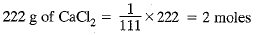1 mole of CaCl2 gives 3 moles of ion
2 moles of CaCl2 gives 6 moles of ion
= 6 x 6.022 x 1023 ions
= 36.132 x 1023 ions = 3.6132 x 1024 ionsQ.27. The difference in the mass of 100 moles each of sodium atoms and sodium ions is 5.48002 g.
Compute the mass of an electron.
Ans.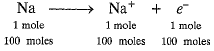100 moles of electrons weigh = 5.48002 g
100 x 6.022 x 1023 electrons weigh = 5.48002 g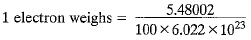= 0.91 x 10-25g = 9.1 x 10-26g = 9.1 x 10-29kg
It is not the real mass of electron.

Q.28. Cinnabar (HgS) is a prominent ore of mercury. How many grams of mercury are present in 225 g of pure HgS? Molar mass of Hg and S are 200.6 g mol-1 and 32 g mol-1 respectively.
Ans.
Molar mass of HgS = 200.6 + 32
= 232.6 g mol-1
232.6 g of HgS contains 200.6 g of pure Hg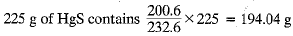Q.29. The mass of one steel screw is 4.11 g. Find the mass of one mole of these steel screws. Compare this value with the mass of the Earth (5.98 x 1024 kg). Which one of the two is heavier and by how many times?
Ans.
Mass of 1 steel screw = 4.11 g
Mass of 6.022 x 1023 steel screws
= 4.11 x 6.022 x 1023 g = 2.475 x 1024 g
= 2.475 x 1021 kg
Therefore, mass of one mole of screws
= 2.475 x 1021 kg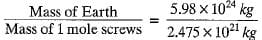= 2.4 x 103
Mass of the earth is 2400 times the mass of 1 mole of screws.

Q.30. A sample of vitamin C is known to contain 2.58 x 1024 oxygen atoms. How many moles of oxygen atoms are present in the sample?
Ans.
Number of moles of oxygen atoms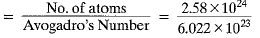= 4.28 moles.

Q.31. Raunak took 5 moles of carbon atoms in a container and Krish also took 5 moles of sodium atoms in another container of the same weight.
(a) Whose container is heavier?
(b) Whose container has more number of atoms?
Ans.
(a) 1 mole of carbon atoms = 12 g
5 moles of carbon atoms = 12 x 5 = 60 g
1 mole of Na = 23 g
5 moles of Na = 23 x 5 = 115 g
Thus, Krish’s container is heavier than that of Raunak.
(b) Both the containers have same number of atoms as both have the same number of moles and both elements are monoatomic.

Q.32. Fill in the missing data in the Table.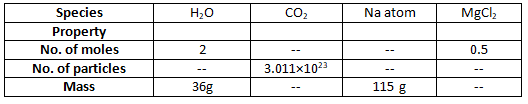Ans. 1 mole of H2O = 1 x 2 + 16 = 18 g
2 moles of H20 = 2 x 18 = 36 g
1 mole of H20 contains 6.022 x 1023 molecule
2 moles of H20 contains 2 x 6.022 x 1023 = 12.044 x 1023 molecules
1 mole of CO2 = 12 + 2 + 16 = 44 g
0.5 mole of CO2 = 44 x 0.5 = 22 g
1 mole of CO2 contains 6.022 x 1023 molecules
0.5 mole of CO2 will contain 0.5 x 6.022 x 1023 = 3.011 x 1023 molecules
1 mole of Na = 23 g
5 moles of Na = 23 x 5 = 115 g
1 mole of Na = 6.022 x 1023 atoms
5 moles of Na = 5 x 6.022 x 1023 = 30.110 x 1023 atoms
1 mole of MgCl2 = 24 + 2 x 35.5 = 24 + 71 = 95 g
0.5 mole of MgCl= 0.5 x 95 = 47.5 g
1 mole of MgCl2 = 6.022 X 1023
0.5 mole of MgCl2 = 0.5 x 6.022 x 1023 = 3.011 x 1023 formula units.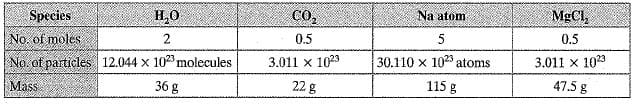Q.33. The visible universe is estimated to contain 1022 stars. How many moles of stars are present in the visible universe?
Ans. Number of moles of stars =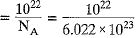= 0.167 x 10-1 = 0.0167 mole.

Q.34. What is the SI prefix for each of the following multiples and sub-multiples of a unit?
(a) 103
(b) 10-1
(c) 10-
(d) 10-6
(e) 10-9
(f) 10-12
Ans.
(a) kilo
(b) deci
(c) centi
(d) micro
(e) nano
(f) pico

Q.35. Express each of the following in kilograms:
(a) 5.84 x 10-3 mg
(b) 58.34 g
(c) 0.584 g
(d) 5.873 x 10-21g
Ans.
(a) 5.84 x 10-3 mg x 10-6 = 5.84 x 10-9 kg [∵ 1 mg = 10-3 g = 10-6 kg]
(b) 58.34 g x 10-3 kg = 5.834 x 10-2 kg [∵ 1 g = 10-3 kg]
(c) 0.584 g = 0.584 x 10-3 kg = 5.84 x 10-4 kg
(d) 5.873 x 10-21 g x 10-3 = 5.873 x 10-24 kg

Q.36. Compute the difference in masses of 103 moles each of magnesium atoms and magnesium ions. (Mass of an electron = 9.1 x 10-31 kg)
Ans.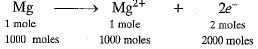1 electron weighs = 9.1 x 10-31 kg
∴ 2000 x 6.022 x 1023 electrons weigh
= 9.1 x 10-31 x 2000 x 6.022 x 1023 kg
= 109.6004 x 10-5 kg = 1.096004 x 10-3 kg

Q.37. Which has more number of atoms? 100 g of N2 or 100 g of NH3
Ans.
1 mole of N2 - 28 g
28 g of N2 = 1 mole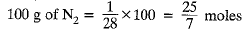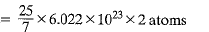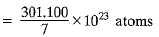= 43.01 x 1023 atoms
= 4.30 x 1024 atoms
1 mole of NH3 = 17 g
17 g of NH3 = 1 mole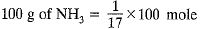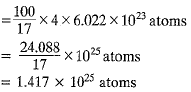Therefore, 100 g of NH3 contains more number of atoms.

Q.38. Compute the number of ions present in 5.85 g of sodium chloride.
Ans.
NaCl → Na+ + Cl-
1 mole of NaCl = 23 + 35.5 = 58.5 g
58.5 g of NaCl = 1 mole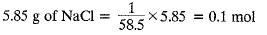1 mole of NaCl gives 2 moles of ions
0.1 mol of NaCl gives 2 x 0.1 = 0.2 mol
= 0.2 x 6.022 x 1023 ions = 1.2044 x 1023 ions

Q.39. A gold sample contains 90% of gold and the rest copper. How many atoms of gold are present in one gram of this sample of gold?
Ans.
Amount of gold in 1 g of 90% pure gold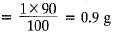1 mole of Au = 197 g1 mole of Au = 6.022 x 1023 atoms
197 g of gold contains 6.022 x 1023 atoms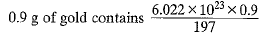= 2.75 x 1021 atoms

Q.40. What are ionic and molecular compounds? Give examples.
Ans.
Ionic compounds are those compounds which are solid and form ions in aqueous solution, have high melting and boiling points, do not conduct electricity in solid state but conduct electricity in molten state or in aqueous solution, e.g. NaCl, KCl, MgO, CaO, etc Molecular compounds may be solids, liquids or gases, do not form ions in aqueous solution, have low melting and boiling points, do not conduct electricity e.g. CH4, CCl4, NH3, PH3, etc.

Q.41. Compute the difference in masses of one mole each of aluminium atoms and one mole of its ions. (Mass of an electron is 9.1 x 10-28 g).
Which one is heavier?
Ans.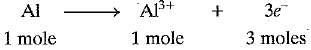Mass of 1 electron = 9.1 x 10-28 g
Mass of 3 x 6.022 x 1023 electrons
= 9.1 x 10-28 x 3 x 6.022 x 1023
Mass of 3 moles of electrons = 164.400 x 10-5 g
= 0.00164 g
Molar mass of Al3+ ions = 27 - 0.00164 g
= 26.9984 g mol-1
Difference in mass between Al and Al3+=0.00164 g
Al atom is heavier than Al3+.

Q.42. A silver ornament of mass ‘m’ gram is polished with gold equivalent to 1% of the mass of silver. Compute the ratio of the number of atoms of gold and silver in the ornament.
Ans.
Mass of silver in the ornament = m gram
Mass of gold in the ornament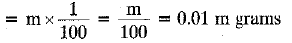108 g of Ag contains 6,022 x 1023 atoms
m gram of Ag contain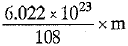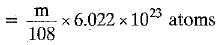197 g of Au contains 6.022 x 1023 atoms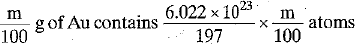Ratio of number of atoms of gold and silver= Au : Ag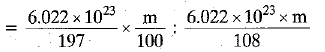= 108 : 19700 = 1 : 182.41

Q.43. A sample of ethane (C2H6) gas has the same mass as 1.5 x 1020 molecules of methane (CH4). How many C2H6 molecules does the sample of gas contain?
Ans.
1 mole of CH4 = 16 g
1 mole of CH4 contains 6.022 x 1023 molecules 6.022 x 1023 molecules of CH4 has mass = 16 g
1.5 x 1020 molecules of CH4 has mass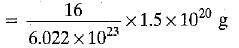Now, 1 mole of C2H6 = 30 g
1 mole of C2H6 = 6.022 x 1023 molecules
30 g of C2H6 contains 6.022 x 1023 moles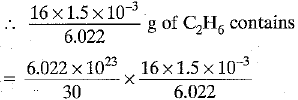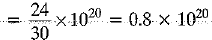= 8 x 1019 molecules

Q.44. Fill in the blanks:
(a) In a chemical reaction, the sum of the masses of the reactants and products remains unchanged. This is called_______.
(b) A group of atoms carrying a fixed charge on them is called_______.
(c) The formula unit m ass of Ca3(PO4)2 is _______ . [Ca = 40 u, P = 31 u, O = 16 u]
(d) Formula of sodium carbonate is _______. and that of ammonium sulphate is______.

Ans. (a) Law of conservation of mass
(c) 40 x 3 + 2 x 31 + 8 x 16
= 120 + 62 + 128 = 310 u
(d) Na2CO3; (NH4)2SO

Q.45. Complete the following crossword puzzle by using the name of the chemical elements. Use the data given in Table.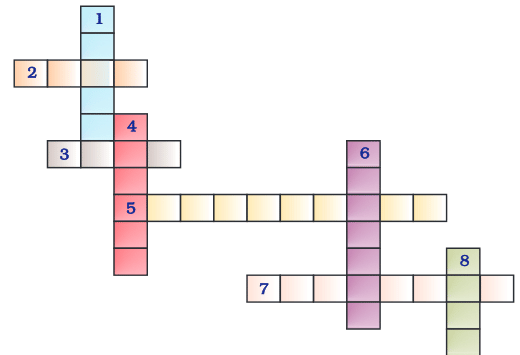Across2. The element used by Rutherford during his a-scattering experiment (4)3. An element which forms rust on exposure to moist air (4)
5. A very reactive non-metal stored under water (10)
7. Zinc metal, when treated with dilute hydrochloric acid, produces a gas of this element which when tested with burning splinter produces a pop sound. (8)
Down
1. A white lustrous metal used for making ornaments and which tends to get tarnished black in the presence of moisture (6)
4. Both brass and bronze are alloys of the element (6)
6. The metal which exists in the liquid state at room temperature (7)
8. An element with symbol Pb (4)
Ans.
> Across :
2. GOLD
3. IRON
5. PHOSPHORUS
7. HYDROGEN
> Down :
1. SILVER
4. COPPER
6. MERCURY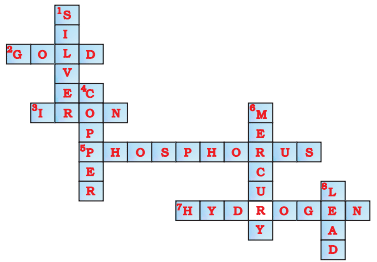Q.46. (a) In the given crossword puzzle, names of 11 elements are hidden. The symbols of these elements are given below. Complete the puzzle.
1. C
2. H
3. Ar
4. O
5. Xe
6. N
7. He
8. F
9. Kr
10. Rn
11. Ne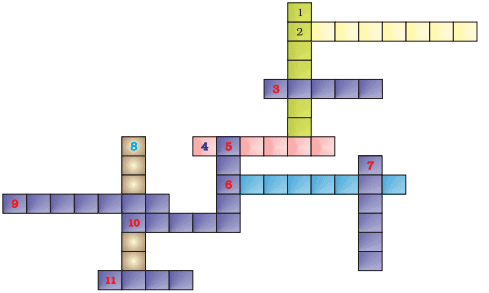(b) Identify the total number of inert gases, their names and symbols from this crossword puzzle.
Ans.
(a)
1. Cl-CHLORINE
2. H-HYDROGEN
3. Ar-ARGON
4. O-OXYGEN
5. Xe-XENON
6. N-NITROGEN
7. He-HELIUM
8. F-FLUORINE
9. Kr-KRYPTON
11. Ne-NEON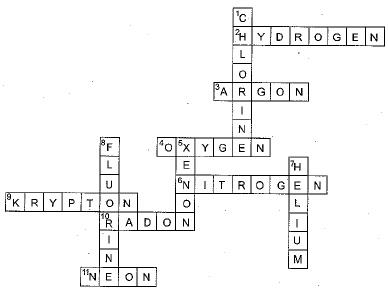(b) Helium (He), Neon (Ne), Argon (Ar), Krypton (Kr), Xenon (Xe) and Radon (Rn) are six inert gases.

Q.47. Write the formulae for the following and calculate the molecular mass for each one of them.
(a) Caustic potash
(b) Baking powder
(c) Lime stone
(d) Caustic soda
(e) Ethanol
(f) Common salt
Ans.
(a) Caustic potash is KOH, Molecular mass
= 39 + 16 + 1 = 56 u
(b) Baking powder is NaHC03, Molecular mass
= 23 + 1 + 12 + 48 - 84 u
(c) Lime stone is CaC03, Molecular mass
= 40 + 12 + 48 - 100 u
(d) Caustic soda is NaOH, Molecular mass
= 23 + 16 + 1 = 40 u
(e) Ethanol is C2H5OH, Molecular mass
= 24 + 5 + 16 + 1 = 46 u
(f) Common salt is NaCl, Molecular mass
= 23 + 35.5 = 58.5 u

Q.48. In photosynthesis, 6 molecules of carbon dioxide combine with an equal number of water molecules through a complex series of reactions to give a molecule of glucose having a molecular formula C6H12O6. How many grams of water would be required to produce 18 g of glucose? Compute the volume of water so consumed, assuming the density of water to be 1 g cm-3.
Ans.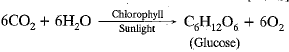Molar mass of C6H12O
= 6 x 12 + 12 x 1 + 6 x 16
= 72 + 12 + 96 = 180 g mol-1
180 g of C6H12O6 needs 108 g of H20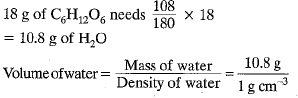Volume of water = 10.8 cm3

The document NCERT Exemplar: Atoms and Molecules | Science Class 9 is a part of the Class 9 Course Science Class 9.
All you need of Class 9 at this link: Class 9

## Science Class 9

66 videos|355 docs|97 tests

## FAQs on NCERT Exemplar: Atoms and Molecules - Science Class 9

 1. What is the difference between atoms and molecules?Ans. Atoms are the smallest units of matter that retain the properties of an element, while molecules are formed when two or more atoms chemically combine and are held together by chemical bonds. In simpler terms, atoms are the building blocks of molecules.
 2. How do atoms and molecules relate to the study of chemistry?Ans. Atoms and molecules are the fundamental entities that form the basis of chemistry. The study of atoms and molecules helps us understand the composition, structure, and behavior of matter. It allows us to delve into the world of chemical reactions, bonding, and the properties of different substances.
 3. What are some examples of molecules?Ans. There are numerous examples of molecules. For instance, water (H2O), carbon dioxide (CO2), oxygen (O2), glucose (C6H12O6), and methane (CH4) are all molecules. These molecules consist of different combinations of atoms of various elements.
 4. How are atoms and molecules related to the concept of conservation of mass?Ans. The concept of conservation of mass states that mass is neither created nor destroyed in a chemical reaction. Atoms and molecules are crucial in understanding this concept because during a chemical reaction, atoms are rearranged and combined to form new molecules. However, the total number of atoms on the reactant side must be equal to the total number of atoms on the product side, ensuring the conservation of mass.
 5. Can atoms be broken down into smaller particles?Ans. Yes, atoms can be broken down into even smaller particles. Atoms consist of a dense nucleus composed of protons and neutrons, which are surrounded by electrons. The nucleus can be further broken down into subatomic particles such as quarks and gluons. However, breaking down an atom into these subatomic particles requires immense energy and is conducted in high-energy particle accelerators.

## Science Class 9

66 videos|355 docs|97 testsExplore Courses for Class 9 examSignup to see your scores go up within 7 days! Learn & Practice with 1000+ FREE Notes, Videos & Tests.
10M+ students study on EduRev
Track your progress, build streaks, highlight & save important lessons and more!
Related Searches

,

,

,

,

,

,

,

,

,

,

,

,

,

,

,

,

,

,

,

,

,

;